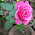MA6451 Probability and Random Processes syllabus for 4th sem ECE - Reg 2013 - Anna University Internal marks 2018
ANNA UNIVERSITY PRP SYLLABUS
4TH SEM ECE
REGULATION 2013

OBJECTIVES:
To provide necessary basic concepts in probability and random processes for applications such as random signals, linear systems etc in communication engineering.

UNIT I RANDOM VARIABLES
Discrete and continuous random variables – Moments – Moment generating functions – Binomial,Poisson, Geometric, Uniform, Exponential, Gamma and Normal distributions.

UNIT II TWO - DIMENSIONAL RANDOM VARIABLES
Joint distributions – Marginal and conditional distributions – Covariance – Correlation and Linear regression – Transformation of random variables.

UNIT III RANDOM PROCESSES
Classification – Stationary process – Markov process - Poisson process – Random telegraph process.

UNIT IV CORRELATION AND SPECTRAL DENSITIES
Auto correlation functions – Cross correlation functions – Properties – Power spectral density – Cross spectral density – Properties.

UNIT V LINEAR SYSTEMS WITH RANDOM INPUTS
Linear time invariant system – System transfer function – Linear systems with random inputs – Auto correlation and Cross correlation functions of input and output.

TOTAL (L:45+T:15): 60 PERIODS

OUTCOMES:
The students will have an exposure of various distribution functions and help in acquiring skills in handling situations involving more than one variable. Able to analyze the response of random inputs to linear time invariant systems.

TEXT BOOKS:
1. Ibe.O.C., “Fundamentals of Applied Probability and Random Processes", Elsevier, 1st Indian Reprint, 2007.
2. Peebles. P.Z., "Probability, Random Variables and Random Signal Principles", Tata Mc Graw Hill,4th Edition, New Delhi, 2002.

REFERENCES:
1. Yates. R.D. and Goodman. D.J., "Probability and Stochastic Processes", 2nd Edition, Wiley India Pvt. Ltd., Bangalore, 2012.
2. Stark. H., and Woods. J.W., "Probability and Random Processes with Applications to Signal Processing", 3rd Edition,Pearson Education, Asia, 2002.
3. Miller. S.L. and Childers. D.G., "Probability and Random Processes with Applications to Signal Processing and Communications", Academic Press, 2004.
4. Hwei Hsu, "Schaum‟s Outline of Theory and Problems of Probability, Random Variables and Random Processes", Tata Mc Graw Hill Edition, New Delhi, 2004.
5. Cooper. G.R., Mc Gillem. C.D., "Probabilistic Methods of Signal and System Analysis", 3rd Indian Edition, Oxford University Press, New Delhi, 2012.

1.